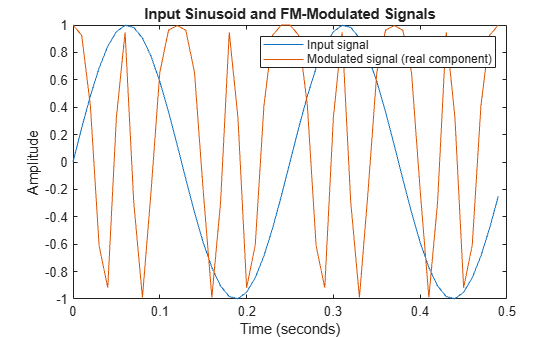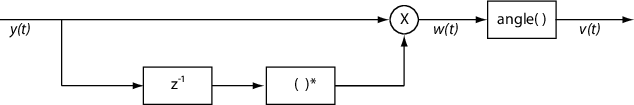# comm.FMDemodulator

Demodulate baseband FM signal

## Description

The `comm.FMDemodulator` System object™ demodulates a baseband FM signal.

To demodulate a baseband FM signal:

1. Create the `comm.FMDemodulator` object and set its properties.

2. Call the object with arguments, as if it were a function.

## Creation

### Syntax

``fmdemodulator = comm.FMDemodulator``
``fmdemodulator = comm.FMDemodulator(Name,Value)``
``fmdemodulator = comm.FMDemodulator(fmmodulator)``

### Description

example

````fmdemodulator = comm.FMDemodulator` creates an FM demodulator System object.```
````fmdemodulator = comm.FMDemodulator(Name,Value)` sets properties using one or more name-value arguments. For example, `'Samplerate',400e3` specifies a sample rate of 400 kHz.```

example

````fmdemodulator = comm.FMDemodulator(fmmodulator)` sets properties based on the configuration of the input `comm.FMModulator` System object, `fmmodulator`.```

## Properties

expand all

Unless otherwise indicated, properties are nontunable, which means you cannot change their values after calling the object. Objects lock when you call them, and the `release` function unlocks them.

If a property is tunable, you can change its value at any time.

Sample rate of the input signal in Hz, specified as a positive scalar. This property specifies the sample rate at the output of a modulator or at the input of a demodulator. The sample rate must be greater than twice the frequency deviation (that is, `SampleRate` > 2×`FrequencyDeviation`).

Data Types: `double`

Peak deviation of the output signal frequency in Hz, specified a positive scalar. The frequency deviation must be less than half the sample rate (that is, `FrequencyDeviation` < `SampleRate`/2).

The system bandwidth is BT = 2×(`FrequencyDeviation` + BM), where BM is the message bandwidth in Hz. For more information, see the Algorithms section.

Data Types: `double`

## Usage

### Syntax

``outsig = fmdemodulator(insig)``

### Description

example

````outsig = fmdemodulator(insig)` demodulates a baseband FM signal and outputs message data.```

### Input Arguments

expand all

Baseband FM signal, specified as a scalar or column vector.

Data Types: `double` | `single` | `fi`
Complex Number Support: Yes

### Output Arguments

expand all

Message data, returned as a scalar or column vector of the same data type and size as the input `insig`.

## Object Functions

To use an object function, specify the System object as the first input argument. For example, to release system resources of a System object named `obj`, use this syntax:

`release(obj)`

expand all

 `step` Run System object algorithm `release` Release resources and allow changes to System object property values and input characteristics `reset` Reset internal states of System object

## Examples

collapse all

Play back an audio file after applying FM modulation and demodulation by using System objects to process the data in streaming mode.

Load the audio file `guitartune.wav` by using an audio file reader System object™.

```audiofilereader = dsp.AudioFileReader('guitartune.wav', ... 'SamplesPerFrame',4410);```

Create an audio device writer System object for audio playback.

`audioplayer = audioDeviceWriter;`

Create default FM modulator and demodulator System objects.

```fmmod = comm.FMModulator; fmdemod = comm.FMDemodulator;```

Read the audio data, FM-modulate the audio data, FM-demodulate the FM-modulated signal, and play back the demodulated signal (`z`).

```while ~isDone(audiofilereader) x = audiofilereader(); y = fmmod(x); z = fmdemod(y); audioplayer(z); end```

Modulate and demodulate a sinusoidal signal. Plot the demodulated signal and compare it to the original signal.

Initialize parameters for the example.

```fs = 100; % Sample rate (Hz) ts = 1/fs; % Sample period (s) fd = 25; % Frequency deviation (Hz)```

Create a sinusoidal signal with a duration of 0.5 s and frequency of 4 Hz.

```t = (0:ts:0.5-ts)'; x = sin(2*pi*4*t);```

Create an FM modulator System object, setting the sample rate and frequency deviation. Then, create an FM demodulator System object, using the FM modulator configuration to set the demodulator properties.

```fmmodulator = comm.FMModulator( ... 'SampleRate',fs, ... 'FrequencyDeviation',fd); fmdemodulator = comm.FMDemodulator(fmmodulator);```

FM-modulate the signal and plot the real component of the complex signal. The frequency of the modulated signal changes with the amplitude of the input signal.

```y = fmmodulator(x); plot(t,[x real(y)]) title('Input Sinusoid and FM-Modulated Signals') xlabel('Time (seconds)'); ylabel('Amplitude') legend('Input signal','Modulated signal (real component)')```Demodulate the FM-modulated signal.

`z = fmdemodulator(y);`

Plot the original and demodulated signals. The demodulator output signal exactly aligns with the original signal.

```plot(t,x,'r',t,z,'ks') legend('Original signal','Demodulated signal') xlabel('Time (s)') ylabel('Amplitude')```Create an FM demodulator System object™ from an FM modulator System object. Modulate and demodulate audio data loaded from a file and compare the spectrum for the demodulated data and the input data.

Initialize parameters for the example.

```fd = 50e3; % Frequency deviation (Hz) fs = 300e3; % Sample rate (Hz)```

Create an FM modulator System object.

```mod = comm.FMModulator( ... 'FrequencyDeviation',fd, ... 'SampleRate',fs);```

Create an FM demodulator object by using the modulator to configure it.

`demod = comm.FMDemodulator(mod);`

Verify that the properties are identical in the two System objects.

`mod`
```mod = comm.FMModulator with properties: SampleRate: 300000 FrequencyDeviation: 50000 ```
`demod`
```demod = comm.FMDemodulator with properties: SampleRate: 300000 FrequencyDeviation: 50000 ```

Load audio data into the workspace.

```S = load('handel.mat'); data = S.y; fsamp = S.Fs;```

Create a spectrum analyzer System object.

```specanalyzer = dsp.SpectrumAnalyzer( ... 'SampleRate',fsamp, ... 'ShowLegend',true);```

FM-modulate and -demodulate the audio data.

```modData = mod(data); demodData = demod(modData);```

Verify that the spectrum plot of the input data (`Channel 1`) aligns with that of the demodulated data (`Channel 2`).

`specanalyzer([data demodData])`## Algorithms

A frequency-modulated passband signal, Y(t), is given as

`$Y\left(t\right)=A\mathrm{cos}\left(2\pi {f}_{\text{c}}t+2\pi {f}_{\text{Δ}}{\int }_{0}^{t}x\left(\text{τ}\right)d\text{τ}\right)\text{\hspace{0.17em}},$`

where:

• A is the carrier amplitude.

• fc is the carrier frequency.

• x(τ) is the baseband input signal.

• fΔ is the frequency deviation in Hz.

The frequency deviation is the maximum shift from fc in one direction, assuming |x(τ)| ≤ 1.

A baseband FM signal can be derived from the passband representation by downconverting the passband signal by fc such that

`$\begin{array}{c}{y}_{\text{s}}\left(t\right)=Y\left(t\right){e}^{-j2\pi {f}_{\text{c}}t}=\frac{A}{2}\left[{e}^{j\left(2\text{π}{f}_{\text{c}}t+2\text{π}{f}_{\text{Δ}}{\int }_{0}^{t}x\left(\text{τ}\right)d\text{τ}\right)}+{e}^{-j\left(2\text{π}{f}_{\text{c}}t+2\text{π}{f}_{\text{Δ}}{\int }_{0}^{t}x\left(\text{τ}\right)d\text{τ}\right)}\right]{e}^{-j2\text{π}{f}_{\text{c}}t}\\ =\frac{A}{2}\left[{e}^{j2\text{π}{f}_{\text{Δ}}{\int }_{0}^{t}x\left(\text{τ}\right)d\text{τ}}+{e}^{-j4\text{π}{f}_{\text{c}}t-j2\text{π}{f}_{\text{Δ}}{\int }_{0}^{t}x\left(\text{τ}\right)d\text{τ}}\right]\text{\hspace{0.17em}}.\end{array}$`

Removing the component at -2fc from yS(t) leaves the baseband signal representation, y(t), which is given as

`$y\left(t\right)=\frac{A}{2}{e}^{j2\pi {f}_{\Delta }{\int }_{0}^{t}x\left(\tau \right)d\tau }.$`

The expression for y(t) can be rewritten as $y\left(t\right)=\frac{A}{2}{e}^{j\varphi \left(t\right)}$, where $\varphi \left(t\right)=2\text{π}{f}_{\Delta }{\int }_{0}^{t}x\left(\tau \right)d\tau$. Expressing y(t) this way implies that the input signal is a scaled version of the derivative of the phase, ϕ(t).

To recover the input signal from y(t), use a baseband delay demodulator, as this figure shows.Subtracting a delayed and conjugated copy of the received signal from the signal itself results in this equation.

`$w\left(t\right)=\frac{{A}^{2}}{4}{e}^{j\varphi \left(t\right)}{e}^{-j\varphi \left(t-T\right)}=\frac{{A}^{2}}{4}{e}^{j\left[\varphi \left(t\right)-\varphi \left(t-T\right)\right]}\text{\hspace{0.17em}},$`

where T is the sample period. In discrete terms,

The signal vn is the approximate derivative of ϕn such that vnxn.

 Hatai, I., and I. Chakrabarti. “A New High-Performance Digital FM Modulator and Demodulator for Software-Defined Radio and Its FPGA Implementation.” International Journal of Reconfigurable Computing (December 25, 2011): 1-10. https://doi.org/10.1155/2011/342532.

 Taub, H., and D. Schilling. Principles of Communication Systems. McGraw-Hill Series in Electrical Engineering. New York: McGraw-Hill, 1971, pp. 142–155.